# GSEB Solutions Class 12 Maths Chapter 8 Application of Integrals Ex 8.2

Gujarat Board GSEB Textbook Solutions Class 12 Maths Chapter 8 Application of Integrals Ex 8.2 Textbook Questions and Answers.

## Gujarat Board Textbook Solutions Class 12 Maths Chapter 8 Application of Integrals Ex 8.2

Question 1.
Find the area of the circle 4x2 + 4y2 = 9 which is interior to the parabola x2 = 4y.
Solution:
Area is bounded by the circle 4x2 + 4y2 = 9 and interior of the parabola x2 = 4y.
Putting x2 = 4 in x2 + y2 = $$\frac{9}{4}$$,we get 4y + y2 = $$\frac{9}{4}$$.
or y2 + 16y – 9 = 0
⇒ (2y + 9)(2y – 1) = 0
y = $$\frac{1}{2}$$, – $$\frac{9}{2}$$; y ≠ – $$\frac{9}{2}$$ as y is positive.
∴ y = $$\frac{1}{2}$$.
Radius of the circle = $$\frac{3}{2}$$
Area of the region required = Area of the region OPRQ
= (area of the region OPQ) + (area of the region PQR)
= 2 × area of the region OPT + 2 × area of the region TPQ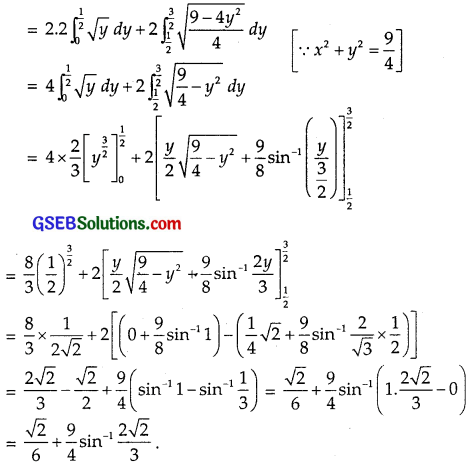Question 2.
Find the area bounded by the curve (x – 1)2 + y2 = 1 and x2 + y2 = 1.
Solution:
Given circles are
x2 + y2 = 1 ………….. (1)
and (x – 1)2 + y2 = 1 ……………….. (2)
Centre of (1) is O (0, 0) and radius = 1.
Both these circles are symmetrical about x-axis.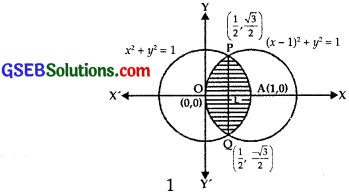Solving (1) and (2) we get,
– 2x + 1 = 0 ⇒ x = $$\frac{1}{2}$$.
Then, y2 = 1 – ($$\frac{1}{2}$$)2 = $$\frac{3}{4}$$ ⇒ y = ±$$\frac{\sqrt{3}}{2}$$.
∴ The points of intersection are P($$\frac{1}{2}$$, $$\frac{\sqrt{3}}{2}$$) and Q($$\frac{1}{2}$$, – $$\frac{\sqrt{3}}{2}$$).
It is clear from the figure that the shaded portion is the region whose area is required.
Required area = area OQAPO
= 2 × area of the region CLAP
= 2 × (area of the region OLPO + area of the region LAPL)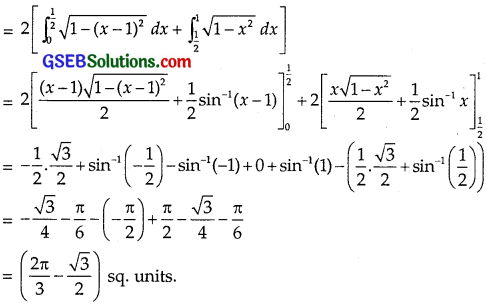Question 3.
Find the area of the region bounded by the curve y = x2 + 2 and the line y = x, x = 0 and x = 3.
Solution:
Equation of the parabola is
y = x2 + 2
or x2 = (y – 2)
Its vertex is (0, 2). Axis is y-axis.
Boundary lines are
y = x, x = 0 and x = 3.
Graphs of the curve and lines have been shown in the figure.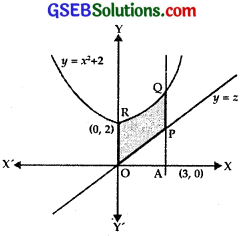Area of the region PQRO
= Area of the region OAQR – Area of region OAP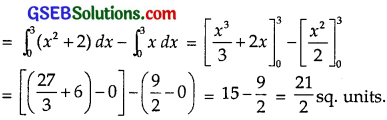Question 4.
Using integration, find the area of the region bounded by a traingle whose vertices are (- 1, 0), (1, 3) and (3, 2).
Solution:
The points A(- 1, 0), B(1, 3) and C(3, 2) are plotted and joined.Area of ∆ ABC = Area of ∆ ABL + Area of trapezium BLMC – Area of ∆ ACM
The equation of the line joining the points (x1, y1) and (x2, y2) isArea of trapezium BCML.
= Area bounded by BC, x-axis x = 1, x = 3.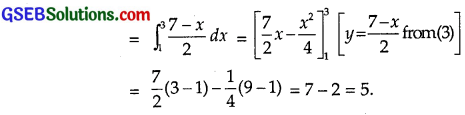Area of ∆ ACM.
(Area bounded by AC, y = $$\frac{x+1}{2}$$ x-axis and x = 3)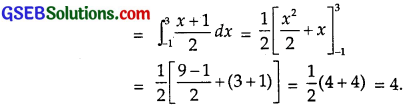From (1),
∴ Area of ∆ ABC = 3 + 5 – 4 = 4 sq.units.Question 5.
Using integration, find the area of the triangular region, whose sides have the equations y = 2x + 1, y = 3x + 1 and x = 4.
Solution:
The given lines are
y = 2x + 1 ………… (1)
y = 3x + 1 …………. (2)
x = 4 ………….. (3)
Subtracting (1) from eq. (2), we get 0 = x,
i.e., x = 0.
Putting x = 0 in eq. (1), we get y = 1.
∴ Lines (2) and (1) intersect at A (0, 1).
Putting x = 4 in eq. (2), we get
y = 12 + 1 = 13.
∴ The lines (2) and (3) intersect at B(4, 13).
Putting x = 4 in eq. (1), we get
y = 8 + 1 = 9.
∴ Lines (1) and (2) intersect at C(4, 9).
Area of ABC
= Area of trapezium OLBA – Area of trapezium OLCA ………… (4)Area of trapezium OLCA
= Area of region bounded of
OC : y = 2x + 1, x = 0 and x = 4 and y = 0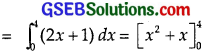= (16 – 0) + (4 – 0) = 20.
Putting these values in (4),
area of ∆ ABC = 28 – 20 = 8 sq.units.Choose the correct answers in the following questions 7 and 8:

Question 6.
Smaller area bounded by the circle x2 + y2 = 4 and the line x + y = 2
(A) 2(π – 2)
(B) π – 2
(C) 2π – 1
(D) 2(π + 2)
Solution:
A circle of radius 2 and centre at O is drawn. The line AB : x + y = 2 we passes through (2, 0) and (0, 2).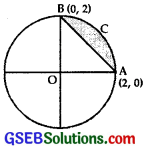Area of the region ACB
= Area of quadrant OAB – Area of ∆ OAB …………. (1)
Now, x2 + y2 = 4
∴ y2 = 4 – x2 or y = $$\sqrt{4-x^{2}}$$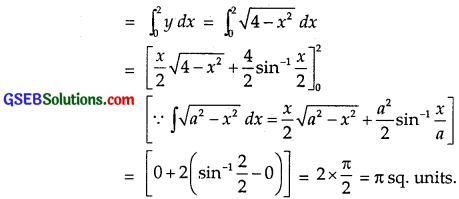Area of ∆ ABC = Area of the region bounded by
AB : x + y = 2 or y = 2 – x
and x = 0, y = 0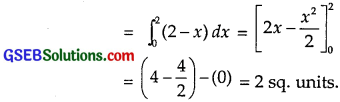Putting these values in (1), we get area of region ACB = π – 2
∴ Part (B) is the correct answer.Question 7.
Area lying between the curves y2 = 4x and y = 2x is
(A) $$\frac{2}{3}$$
(B) $$\frac{1}{3}$$
(C) $$\frac{1}{4}$$
(D) $$\frac{3}{4}$$
Solution:
The curve is y2 = 4x …………… (1)
and the line is y = 2x …………….. (2)
From (1) and (2),
(4x)2 = 4
∴ x(x – 1) = 0
x = 0, x = 1.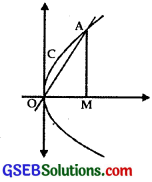Curves (1) and (2) intersect at O(0, 0) and A(1, 2).
∴ Area of region OACO
= Area of region OMACO – Area of OMA ………………. (3)
Now, area of region OMACO
= Area of region bounded by curve OCA
y = $$\sqrt{4x}$$, x-axis and x = 1.Area of ∆ OMA = Area of region bounded by
OA : y = 2x, x = 1 and x-axis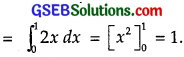Putting these values in (3), we get
are of region OMACO
= $$\frac{4}{3}$$ – 1 = $$\frac{1}{3}$$ sq.units.